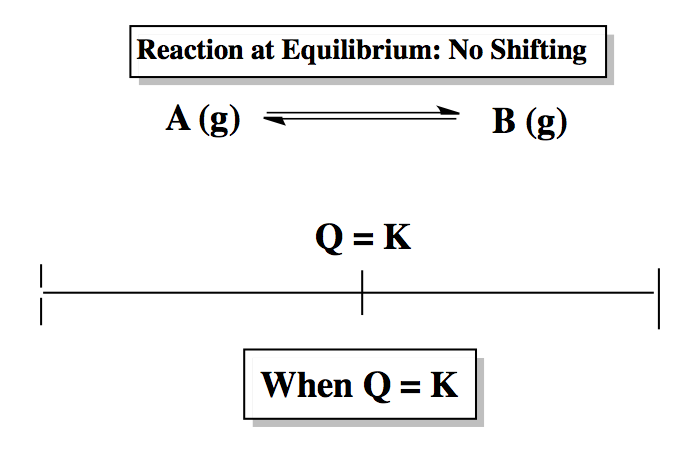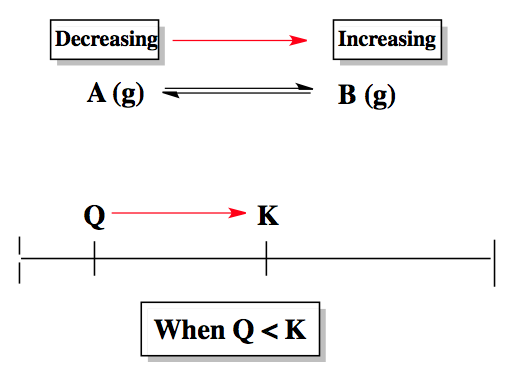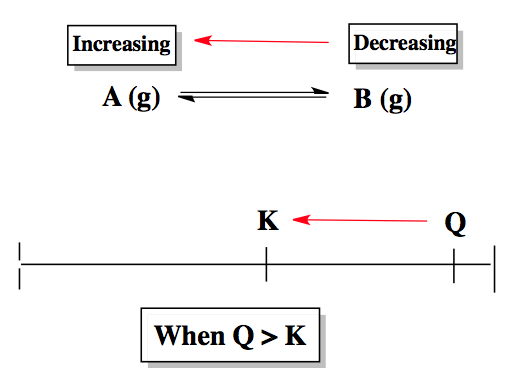Clutch Prep is now a part of Pearson
Ch.14 - Chemical EquilibriumWorksheetSee all chapters

# The Reaction Quotient

See all sections
Sections
Chemical Equilibrium
ICE Chart
Le Chatelier's Principle
The Reaction Quotient
Equilibrium Expressions

The reaction quotient, Q, is used to determine if our chemical reaction is at equilibrium.

###### Equilibrium Determination

Depending on if Q is greater than or less than K our reaction will shift to attain equilibrium by reaching the equilibrium constant K. The direction our reaction shifts determines whether our reactants or products are increasing or decreasing.

Example #1: Q vs. K

If the reaction quotient Q is equal to the equilibrium constant K then our reaction is at equilibrium.When the reaction quotient, Q, is smaller than the equilibrium constant, K, then the reaction shifts in the forward direction to reach equilibrium.When the reaction quotient, Q, is larger than the equilibrium constant, K, then the reaction shifts in the reverse direction to reach equilibrium.Example #2: Determining the Reaction Quotient

Example #3: Determining Equilibrium Concentrations